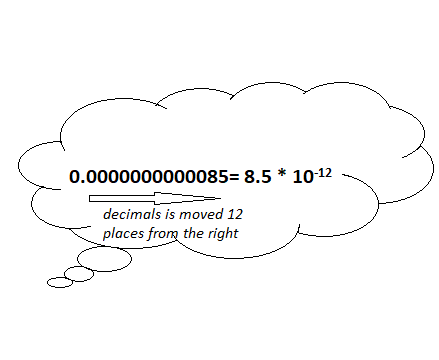# NCERT solutions for class 8 maths chapter 12 exercise 12.2

In this page we have ncert solutions for class 8 maths chapter 12 exercise 12.2 for Exponents and Power.This exercise has questions about Use of Exponents to Express Small Numbers in Standard Form and comparing small vs large numbers. Hope you like them and do not forget to like , social share and comment at the end of the page.

## NCERT solutions for class 8 maths chapter 12 exercise 12.2

Question 1
Express the following numbers in standard form.
1. 0.0000000000085
2. 0.00000000000942
3. 6020000000000000
4. 0.00000000837
5. 31860000000

1. 0.0000000000085 =8.5 ×10-122. 0.00000000000942 =9.42×10-12
3. 6020000000000000 =6.02×1015
4. 0.00000000837 =8.37 ×10-9
5. 31860000000 =3.186×1010

Question 2
Express the following numbers in usual form.
1. 3.02 x 10-6
2. 4.5 x 104
3. 3 x 10-8
4. 1.0001 x 109
5. 5.8 x 1012
6. 3.61492 x 106

1. 3.02 x 10-6 = .00000302
2. 4.5 x 104 =45000
3. 3 x 10-8 =.00000003
4. 1.0001 x 109 =1000100000
5. 5.8 x 1012 =5800000000000
6. 3.61492 x 106=3614920

Question 3
Express the number appearing in the following statements in standard form.
(i) 1 micron is equal to 1/1000000 m.
(ii) Charge of an electron is 0.000, 000, 000, 000, 000, 000, 16 coulomb.
(iii) Size of bacteria is 0.0000005 m
(iv)  Size of a plant cell is 0.00001275 m
(v) Thickness of a thick paper is 0.07 mm

(i)1/1000000
=1×10-6
(ii) 0.000, 000, 000, 000, 000, 000, 16
=1.6 ×10-19
(iii) 0.0000005
=5 ×10-7
(iv)0.00001275
=1.275 ×10-5
(v) 0.07
=7×10-2

Question 4
In a stack there are 5 books each of thickness 20 mm and 5 paper sheets each of thickness 0.016 mm. What is the total thickness of the stack?

Total thickness
=5×20  + 5X.016
=100  + 5× 1.6 ×10-2
=100  + .08
=100.08 mm

## Summary

1. NCERT solutions for class 8 maths chapter 12 exercise 12.2 has been prepared by Expert with utmost care. If you find any mistake.Please do provide feedback on mail. You can download the solutions as PDF in the below Link also
2. This chapter 12 has total 2 Exercise 12.1 and 12.2. This is the last exercise in the chapter.You can explore previous exercise of this chapter by clicking the link below